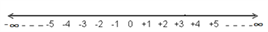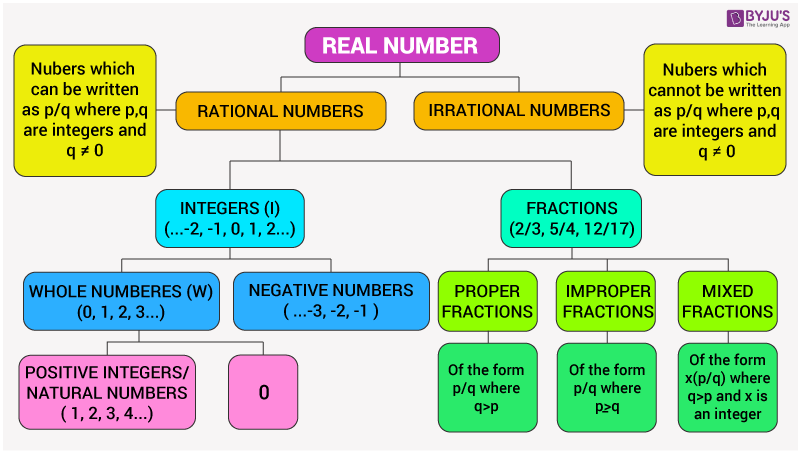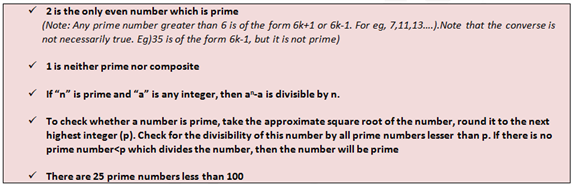# Classification Of Numbers

Knowing the classification of numbers and their properties properly are very crucial to solve the CAT quantitative aptitude section easily. In this article, the classification of numbers are explained thoroughly and all the related terms and definitions are mentioned along with solved examples.

Introduction: Classification Of Numbers

The broadest classification of numbers is into real numbers and complex numbers. Real numbers are the ones which can be represented on the number line and complex numbers are those which cannot be represented on a number line.

1) Real numbers can be classified into rational numbers and irrational numbers. A clear classification of real numbers is shown in the table

2) Complex numbers are of the form p+qi where “p” is the real part and “qi” is the imaginary part. “i” is defined as √-1.

• Number Line:Note: The number line goes on to infinity in both directions.

Left side of zero➜ negative integers;

Right side of zero➜ positive integers;

Zero + positive integers➜ whole numbers.• Comparing Integers

Integers can be compared by looking at their positions on the number line. The integer on the right will always be greater than the integer on the left i.e. every positive integer is greater than any negative integer.

Important Definitions Related to Numbers:

1. Natural Numbers

The numbers used for counting are called natural numbers. They are present on the positive side of the number line. They are also called positive integers. The lowest natural number is one.

2. Whole Numbers

When zero is included with the natural numbers, the set of numbers are called whole numbers.

3. Integers

They comprise of natural numbers, negative integers and zero. Zero is neither positive nor negative.

Two integers are said to be opposites if each of the two is from the same distance from the origin. One will have a positive sign and the other will have a negative sign.

4. Prime Numbers And Composite Numbers

Numbers that are divisible by only 1 and itself are called PRIME NUMBERS.

Example: 2, 7, 11, 13.

NOTE: Factors are those numbers which divide a given number completely, that is, without leaving a remainder ( 2 is a factor of 8 whereas, 7 is not a factor of 22)

Numbers that have factors other than itself and unity are called COMPOSITE NUMBERS.

Example: 4, 6, 8, 15.Illustration:

For example, to check whether 37 is prime, take the approximate square root of 37=6 Check if there is a prime number below 6 which divides 37.

None of 2, 3 and 5 divides 37. Hence, 37 is prime.

5. Relatively Prime Numbers

Numbers whose highest common factor is 1 (i.e the highest common divisor of both the numbers is 1) are said to be relatively prime numbers.

Example: 6 and 13 are relatively prime; since 1 is the highest common factor of these two numbers; similarly 2 and 3, 7 and 9 etc.

6. Odd Numbers And Even Numbers

If “A” is an integer and if there is an integer “N” such that A=2N+1, then A is said to be an odd number.

If there exists an integer N such that A=2N, then A is an even number.

EVEN NUMBERS are those numbers which are divisible by 2 (positive or negative) whereas ODD NUMBERS are those numbers(positive or negative) that are not divisible by 2.

Stay tuned to BYJU’S for latest CAT exam details, notification and updates. Get study materials and interactive video lessons for CAT exam preparation, prepared by best CAT trainers of India.